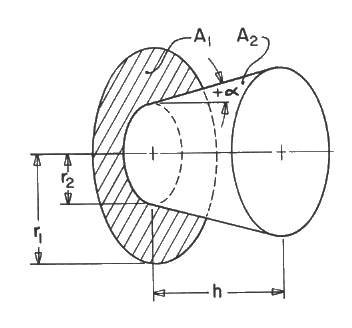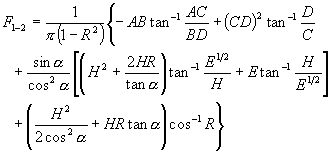° Radiation Configuration Factors C-49.html

# SECTION C Factors From Finite Areas to Finite Areas

## C-49: Annular disk to coaxial truncated cone; cone can be convergent (+α) or divergent (-α).Definitions:
 H = h/r1;  R = r2/r1; A = [H2 + (1 + R + Htanα)2]1/2; B = [H2 + (1 - R - Htanα)2]1/2; C = (1 - R)1/2;  D = (1 + R)1/2;  E = cos2α(1 - R2)

Governing equation:To download a copy of this equation in MS Word 97 Format, click HERE.